# Maharashtra Board 12th Maths Solutions Chapter 6 Line and Plane Ex 6.2

Balbharti 12th Maharashtra State Board Maths Solutions Book Pdf Chapter 6 Line and Plane Ex 6.2 Questions and Answers.

## Maharashtra State Board 12th Maths Solutions Chapter 6 Line and Plane Ex 6.2

Question 1.
Find the length of the perpendicular from (2, -3, 1) to the line $$\frac{x+1}{2}=\frac{y-3}{3}=\frac{z+1}{-1}$$
Solution:
Let PM be the perpendicular drawn from the point P (2, -3, 1) to the line $$\frac{x+1}{2}=\frac{y-3}{3}=\frac{z+1}{-1}$$ = λ …(Say)
The coordinates of any point on the line are given by x = -1 + 2λ, y = 3 + 3λ, z = -1 – λ
Let the coordinates of M be
(-1 + 2λ, 3 + 3λ, -1 – λ) … (1)
The direction ratios of PM are
-1 + 2λ – 2, 3 + 3λ + 3, -1 – λ – 1
i.e. 2λ – 3, 3λ + 6, -λ – 2
The direction ratios of the given line are 2, 3, -1.
Since PM is perpendicular to the given line, we get
2(2λ – 3) + 3(3λ + 6) – 1(-λ – 2) = 0
∴ 4λ – 6 + 9λ + 18 + λ + 2 = 0
∴ 14λ + 14 = 0 ∴ λ = -1.
Put λ = -1 in (1), the coordinats of M are
(-1 – 2, 3 – 3, -1 + 1) i.e. (-3, 0,0).
∴ length of perpendicular from P to the given line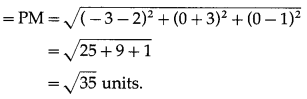Alternative Method:
We know that the perpendicular distance from the point P$$|\bar{\alpha}|$$ to the line $$\bar{r}=\bar{a}+\lambda \vec{b}$$ is given by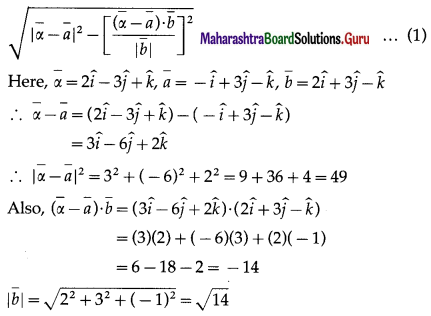Substituting these values in (1), we get
length of perpendicular from P to given line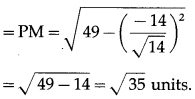Question 2.
Find the co-ordinates of the foot of the perpendicular drawn from the point $$2 \hat{i}-\hat{j}+5 \hat{k}$$ to the line $$\bar{r}=(11 \hat{i}-2 \hat{j}-8 \hat{k})+\lambda(10 \hat{i}-4 \hat{j}-11 \hat{k})$$. Also find the length of the perpendicular.
Solution:
Let M be the foot of perpendicular drawn from the point P ($$2 \hat{i}-\hat{j}+5 \hat{k}$$) on the line
$$\bar{r}=(11 \hat{i}-2 \hat{j}-8 \hat{k})+\lambda(10 \hat{i}-4 \hat{j}-11 \hat{k})$$.
Let the position vector of the point M be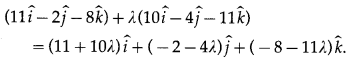Then $$\overline{\mathrm{PM}}$$ = Position vector of M – Position vector of P
= [(11 + 10λ)$$\hat{i}$$ + (-2 – 4λ)$$\hat{j}$$ + -8 – 11λ) $$\hat{k}$$] – (2$$\hat{i}$$ – $$\hat{j}$$ + 5$$\hat{k}$$)
= (9 + 10λ)$$\hat{i}$$ + (-1 – 4λ)$$\hat{j}$$ + (-13 – 11λ)$$\hat{k}$$
Since PM is perpendicular to the given line which is parallel to $$\bar{b}=10 \hat{i}-4 \hat{j}-11 \hat{k}$$,
$$\overline{\mathrm{PM}}$$ ⊥r$$\bar{b}$$ ∴ $$\overline{\mathrm{PM}} \cdot \bar{b}$$ = 0
∴ [(9 + 10λ)$$\hat{i}$$ + ( – 1 – 4λ)$$\hat{j}$$ + (-13 – 11λ)$$\hat{k}$$]-(10$$\hat{i}$$ – 4$$\hat{j}$$ – 11$$\hat{k}$$) = 0
∴ 10(9 +10λ) – 4( -1 – 4λ) – 11( -13 – 11λ) = 0
∴ 90 + 100λ + 4 + 16λ + 143 +121λ = 0
∴ 237λ + 237 = 0
∴ λ = -1
Putting this value of λ, we get the position vector of M as $$\hat{i}+2 \hat{j}+3 \hat{k}$$.
∴ coordinates of the foot of perpendicular M are (1, 2, 3).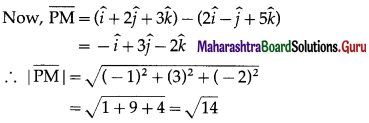Hence, the coordinates of the foot of perpendicular are (1,2, 3) and length of perpendicular = $$\sqrt {14}$$ units.Question 3.
Find the shortest distance between the lines $$\bar{r}=(4 \hat{i}-\hat{j})+\lambda(\hat{i}+2 \hat{j}-3 \hat{k})$$ and $$\bar{r}=(\hat{i}-\hat{j}+2 \hat{k})+\mu(\hat{i}+4 \hat{j}-5 \hat{k})$$
Solution:
We know that the shortest distance between the skew lines $$\bar{r}=\overline{a_{1}}+\lambda \overline{b_{1}}$$ and $$\bar{r}=\overline{a_{2}}+\mu \overline{b_{2}}$$ is given by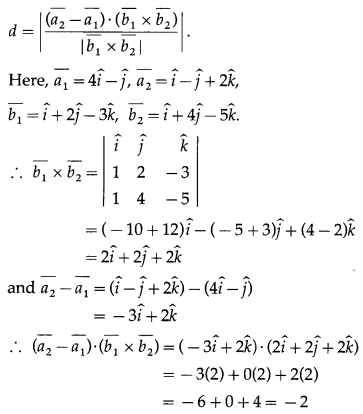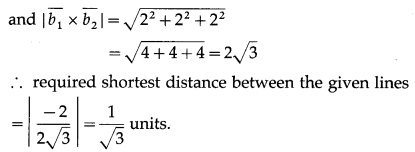Question 4.
Find the shortest distance between the lines $$\frac{x+1}{7}=\frac{y+1}{-6}=\frac{z+1}{1}$$ and $$\frac{x-3}{1}=\frac{y-5}{-2}=\frac{z-7}{1}$$
Solution:
The shortest distance between the lines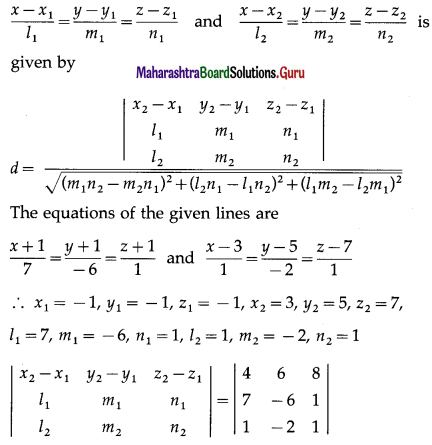= 4(-6 + 2) – 6(7 – 1) + 8(-14 + 6)
= -16 – 36 – 64 = -116
and (m1n2 – m2n1)2 + (l2n1 – l1n2)2 + (l1m2 – l2m1)2
= (-6 + 2)2 + (1 – 7)2 + (-14 + 6)2
= 16 + 36 + 64 = 116
Hence, the required shortest distance between the given lines = $$\left|\frac{-116}{\sqrt{116}}\right|$$ = $$\sqrt{116}$$ = $$2 \sqrt{29}$$ unitsQuestion 5.
Find the perpendicular distance of the point (1, 0, 0) from the line $$\frac{x-1}{2}=\frac{y+1}{-3}=\frac{z+10}{8}$$ Also find the co-ordinates of the foot of the perpendicular.
Solution:
Let PM be the perpendicular drawn from the point (1, 0, 0) to the line $$\frac{x-1}{2}=\frac{y+1}{-3}=\frac{z+10}{8}$$ = λ …(Say)
The coordinates of any point on the line are given by x = -1 + 2λ, y = 3 + 2λ, z = 8 – λ
Let the coordinates of M be
(-1 + 2λ, 3 + 3λ, -1 – λ) …..(1)
The direction ratios of PM are
-1 + 2λ – 2, 3 + 3λ + 3, -1 – λ – 1
i.e. 2λ – 3, 3λ = 6, -λ – 2
The direction ratios of the given line are 2, 3, 8.
Since PM is perpendicular to the given line, we get
2(2λ – 3) + 3(3λ + 6) – 1(-λ – 2) = O
∴ 4λ – 6 + 9λ + 18 + λ + 2 = 0
∴ 14λ + 14 = 0
∴ λ = -1
Put λ in (1), the coordinates of M are
(-1 – 2, 3 – 3, -1 + 1) i.e. (-3, 0, 0).
∴ length of perpendicular from P to the given line
= PM
= $$\sqrt{(-3-2)^{2}+(0+3)^{2}+(0-1)^{2}}$$
= $$\sqrt{(25 + 9 + 1)}$$
= $$\sqrt{35}$$units.
Alternative Method :
We know that the perpendicular distance from the point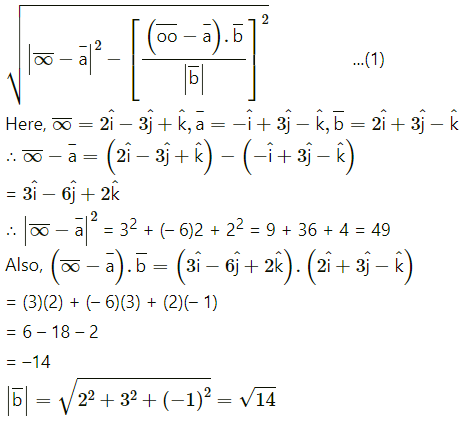Substitutng tese values in (1), w get
length of perpendicular from P to given line
= PM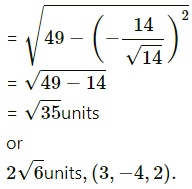Question 6.
A(1, 0, 4), B(0, -11, 13), C(2, -3, 1) are three points and D is the foot of the perpendicular from A to BC. Find the co-ordinates of D.
Solution:
Equation of the line passing through the points (x1, y1, z1) and (x2, y2, z2) is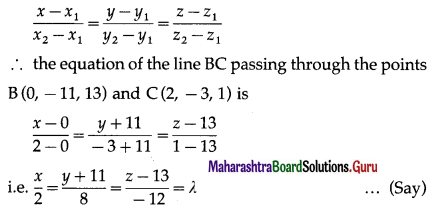AD is the perpendicular from the point A (1, 0, 4) to the line BC.
The coordinates of any point on the line BC are given by x = 2λ, y = -11 + 8λ, z = 13 – 12λ
Let the coordinates of D be (2λ, -11 + 8λ, 13 – 12λ) … (1)
∴ the direction ratios of AD are
2λ – 1, -1λ + 8λ – 0, 13 – 12λ – 4 i.e.
2λ – 1, -11 + 8λ, 9 – 12λ
The direction ratios of the line BC are 2, 8, -12.
Since AD is perpendicular to BC, we get
2(2λ – 1) + 8(-11 + 8λ) – 12(9 – 12λ) = 0
∴ 42λ – 2 – 88 + 64λ – 108 + 144λ = 0
∴ 212λ – 198 = 0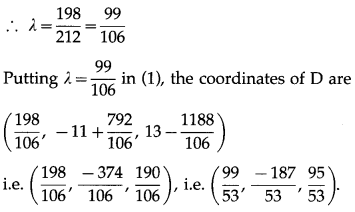Question 7.
By computing the shortest distance, determine whether following lines intersect each other.
(i) $$\bar{r}=(\hat{i}-\hat{j})+\lambda(2 \hat{i}+\hat{k})$$ and $$\bar{r}=(2 \hat{i}-\hat{j})+\mu(\hat{i}+\hat{j}-\hat{k})$$
Solution:
The shortest distance between the lines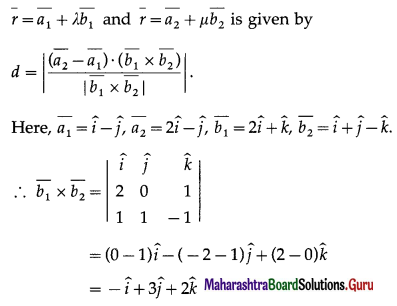Hence, the given lines do not intersect.(ii) $$\frac{x-5}{4}=\frac{y-7}{-5}=\frac{z+3}{-5}$$ and $$\frac{x-8}{7}=\frac{y-7}{1}=\frac{z-5}{3}$$
Solution:
The shortest distance between the lines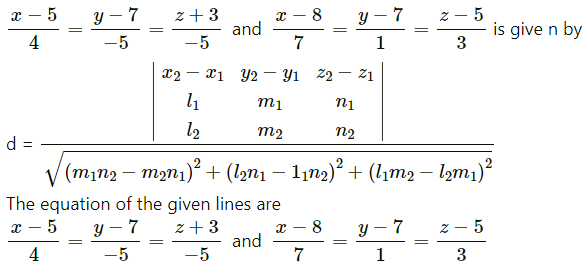∴ x1 = -1, y1 = -1, z1 = -1, x2 = 3, y2 = 5, z2 = 7,
l1 = 7, m1 = -6, n1 = 1, l2 = 1, m2 = -2, n2 = 1
$$\left|\begin{array}{ccc} x_{2}-x_{1} & y_{2}-y_{1} & z_{2}-z_{1} \\ l_{1} & m_{1} & n_{1} \\ l_{2} & m_{2} & n_{2} \end{array}\right|$$ = $$\left|\begin{array}{ccc} 4 & 6 & 8 \\ 4 & -5 & -5 \\ 7 & 1 & 3 \end{array}\right|$$
= 4(- 6 + 2) – 6(7 – 1) + 8(-14 + 6)
= -16 – 36 – 64
= -116
and
(m1n2 – m2n1)2 + (l2n1 – l1n2)2 + (l1m2 – l2m1)2
= (-6 + 2)2 + (1 – 7)2 + (-14 + 6)2
= 16 + 36 + 64
= 116
Hence, the required shortest distance between the given lines
= $$\left|\frac{-116}{\sqrt{116}}\right|$$
= $$\sqrt{116}$$
=$$2 \sqrt{29}$$ units
or
The shortest distance between the lines
= $$\frac{282}{\sqrt{3830}}$$units
Hence, the gives lines do not intersect.Question 8.
If lines $$\frac{x-1}{2}=\frac{y+1}{3}=\frac{z-1}{4}$$ and $$\frac{x-3}{1}=\frac{y-k}{2}=\frac{z}{1}$$ intersect each other then find k.
Solution:
The lines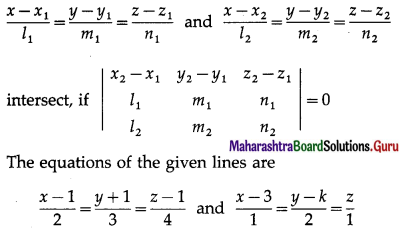∴ x1 = 1, y1 = -1, z1 = 1, x2 = 3, y2 = k, z2 = 0,
l1 = 2, m1 = 3, n1 = 4, l2 = 1, m2 = 2, n2 = 1.
Since these lines intersect, we get
$$\left|\begin{array}{ccc} 2 & k+1 & -1 \\ 2 & 3 & 4 \\ 1 & 2 & 1 \end{array}\right|$$ = 0
∴ 2 (3 – 8) – (k + 1)(2 – 4) – 1 (4 – 3) = 0
∴ -10 + 2(k + 1) – 1 = 0
∴ 2(k + 1) = 11
∴ k + 1 = $$\frac{11}{2}$$
∴ k = $$\frac{9}{2}$$